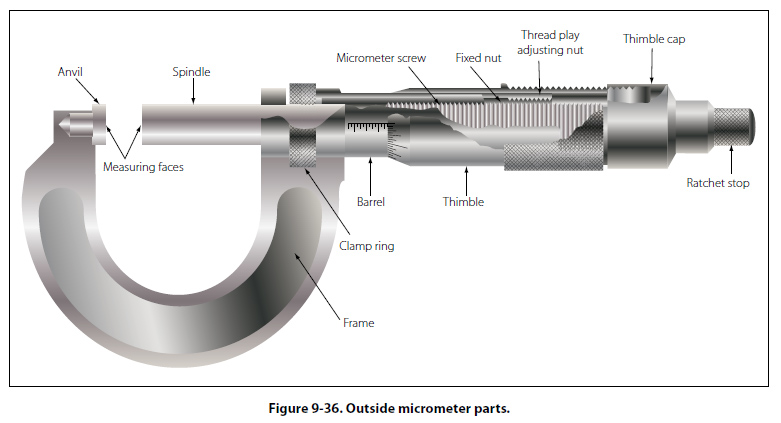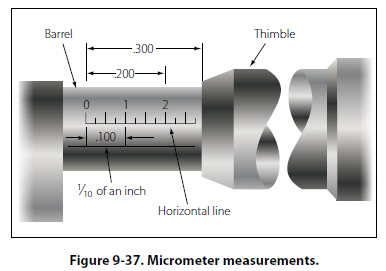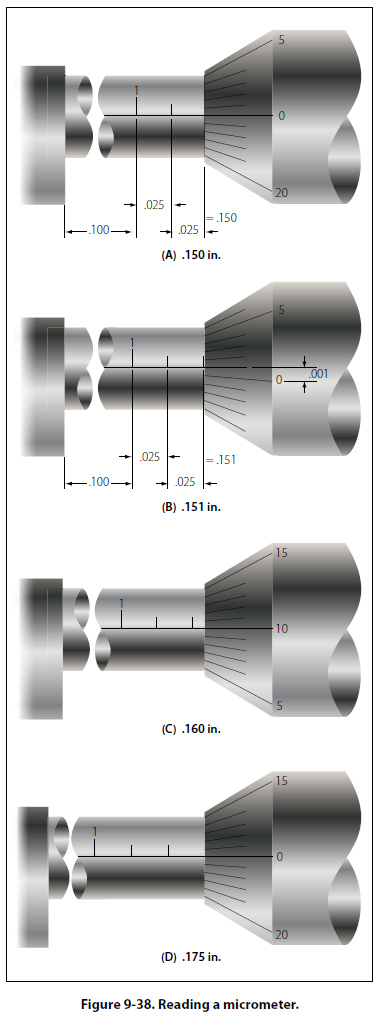Micrometer Parts The fixed parts of a micrometer are the frame, barrel, and anvil. The movable parts of a micrometer are the thimble and spindle. The thimble rotates the spindle which moves in the threaded portion inside the barrel. Turning the thimble provides an opening between the anvil and the end of the spindle where the work is measured. The size of the work is indicated by the graduations on the barrel and thimble. [Figure 9-36]Reading a Micrometer The lines on the barrel marked 1, 2, 3, 4, and so forth, indicate measurements of tenths, or 0.100 inch, 0.200 inch, 0.300 inch, 0.400 inch, respectively. [Figure 9-37]Each of the sections between the tenths divisions (between 1, 2, 3, 4, and so forth) is divided into four parts of 0.025 inch each. One complete revolution of the thimble (from zero on the thimble around to the same zero) moves it one of these divisions (0.025 inch) along the barrel. The bevel edge of the thimble is divided into 25 equal parts. Each of these parts represents one twenty-fifth of the distance the thimble travels along the barrel in moving from one of the 0.025 inch divisions to another. Thus, each division on the thimble represents one onethousandth (0.001) of an inch. These divisions are marked for convenience at every five spaces by 0, 5, 10, 15, and 20. When 25 of these graduations have passed the horizontal line on the barrel, the spindle (having made one revolution) has moved 0.025 inch. The micrometer is read by first noting the last visible figure on the horizontal line of the barrel representing tenths of an inch. Add to this the length of barrel between the thimble and the previously noted number. (This is found by multiplying the number of graduations by 0.025 inch.) Add to this the number of divisions on the bevel edge of the thimble that coincides with the line of the graduation. The total of the three figures equals the measurement. (Figure 9-38 shows several sample readings.)©AvStop Online Magazine                                                                                                                                                      Contact Us              Return To Books